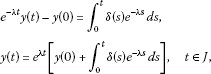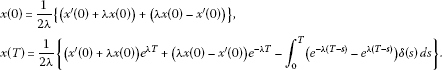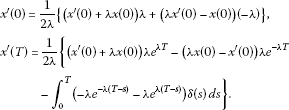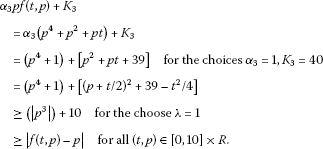# New results on anti-periodic boundary value problems for second-order nonlinear differential equations

## Abstract

This paper is concerned with the solvability of anti-periodic boundary value problems for second-order nonlinear differential equations. By using topological methods, some sufficient conditions for the existence of solution are obtained, which extend and improve the previous results.

MSC: 34B05.

## 1 Introduction

In this paper, we will consider the existence of solutions to second-order differential equations of the type

${x}^{″}\left(t\right)=f\left(t,x\left(t\right),{x}^{\prime }\left(t\right)\right),\phantom{\rule{1em}{0ex}}t\in J=\left[0,T\right],$
(1.1)

subject to the anti-periodic boundary conditions

$x\left(0\right)+x\left(T\right)=0,\phantom{\rule{2em}{0ex}}{x}^{\prime }\left(0\right)+{x}^{\prime }\left(T\right)=0,$
(1.2)

where T is a positive constant and $f:\left[0,T\right]×R×R\to R$ is continuous. Equation (1.1) subject to (1.2) is called an anti-periodic boundary value problem.

Anti-periodic problems have been studied extensively in recent years. For example, anti-periodic boundary value problems for ordinary differential equations were considered in . Also, anti-periodic boundary conditions for impulsive differential equations, partial differential equations and abstract differential equations were investigated in . The methods and techniques employed in these papers involve the use of the Leray-Schauder degree theory [7, 8], the upper and lower solutions [2, 1012], and a fixed point theorem . By using Schauder’s fixed point theorem and lower and upper solutions method, Wang and Shen in  considered the anti-periodic boundary value problem (1.1) and (1.2) when a first-order derivative is not involved explicitly in the nonlinear term f, namely equation (1.1) reduces to

${x}^{″}\left(t\right)=f\left(t,x\left(t\right)\right),\phantom{\rule{1em}{0ex}}t\in J.$
(1.3)

They proved the following theorems.

Theorem 1.1 ([, Theorem 2.2])

Assume there exist constants $0, $l>0$, and functions $p,q,h\in C\left(J,R\right)$ such that

$uf\left(t,u\right)\le p\left(t\right){u}^{2}+q\left(t\right){|u|}^{r}+h\left(t\right)$
(1.4)

for $t\in J$ and $|u|>l$. Further suppose that

${\int }_{0}^{T}{p}^{+}\left(s\right)\phantom{\rule{0.2em}{0ex}}ds<4,$

where ${p}^{+}\left(t\right)=max\left\{p\left(t\right),0\right\}$. Then (1.2) and (1.3) have at least one solution.

Theorem 1.2 ([, Theorem 1.2])

Let γ be a positive constant. Assume there exist a continuous and nondecreasing function $\psi :\left[0,\mathrm{\infty }\right)\to \left(0,\mathrm{\infty }\right)$ and a nonnegative function $p\in C\left(J,R\right)$ with

$|f\left(t,u\right)+{\gamma }^{2}u|\le p\left(t\right)\psi \left(|u|\right)$

for $t\in J$ and $u\in R$. Further suppose that

$\underset{u\to \mathrm{\infty }}{lim sup}\frac{\psi \left(u\right)}{u}
(1.5)

Then (1.2) and (1.3) have at least one solution.

In this paper, we are interested in the existence of a solution to the anti-periodic boundary value problem (1.1) and (1.2). The significant point here is that the right-hand side of (1.1) may depend on ${x}^{\prime }$. The dependence of right-hand side on ${x}^{\prime }$ is naturally seen in many physical phenomena, and we refer the readers to [17, 18] for some nice examples. If there appears ${x}^{\prime }$ in nonlinear term, the relative boundary value problem will be more complicated. Meanwhile, we note equation (1.4) or (1.5) implies that $f\left(t,x\right)$ is at most linear for x, so the problem has not been solved when $f\left(t,x\right)$ is super-linear for x. Motivated by the above two aspects, we devote ourselves to studying the anti-periodic boundary value problem (1.1) and (1.2).

The paper is organized as follows. In Section 2, we reformulate the anti-periodic boundary value problem (1.1) and (1.2) as an equivalent integral equation, which is a widely used technique in the theory of boundary value problem. In Section 3, a general existence result is presented for (1.1) and (1.2). The result provides a natural motivation for the obtention of a priori bounds on solutions and greatly minimizes the proofs of the new results in the following section. The main tool used here is the Leray-Schauder topological degree. In Section 4, some new conditions are presented for (1.1) and (1.2). The new conditions involve linear or quadratic growth constraints on $|f\left(t,p,q\right)|$ in $|q|$.

## 2 Preliminaries

If a function $x\in {C}^{2}\left(J,R\right)$ satisfies equations (1.1) and (1.2), we call x a solution of (1.1) and (1.2). Let ${C}^{1}\left(J,R\right)$ be a Banach space with the norm $\parallel x\parallel =max\left\{{|x|}_{0},{|{x}^{\prime }|}_{0}\right\}$, where ${|x|}_{0}={max}_{t\in J}|x\left(t\right)|$, ${|{x}^{\prime }|}_{0}={max}_{t\in J}|{x}^{\prime }\left(t\right)|$.

Let $\lambda >0$, $\delta \left(t\right)\in C\left(J,R\right)$ and consider the anti-periodic boundary value problem

$\left\{\begin{array}{c}{x}^{″}\left(t\right)-{\lambda }^{2}x\left(t\right)=\delta \left(t\right),\phantom{\rule{1em}{0ex}}t\in J,\hfill \\ x\left(0\right)+x\left(T\right)=0,\phantom{\rule{2em}{0ex}}{x}^{\prime }\left(0\right)+{x}^{\prime }\left(T\right)=0.\hfill \end{array}$
(2.1)

Lemma 2.1 x is a solution of (2.1) if and only if x satisfies

$x\left(t\right)={\int }_{0}^{T}G\left(t,s\right)\delta \left(s\right)\phantom{\rule{0.2em}{0ex}}ds,$
(2.2)

where

$G\left(t,s\right)=\left\{\begin{array}{cc}\frac{{e}^{\lambda \left(t-s\right)}-{e}^{\lambda \left(T-t+s\right)}}{2\lambda \left(1+{e}^{\lambda T}\right)},\hfill & 0\le s

Proof Suppose $x\left(t\right)$ is a solution of (2.1) and denote $D=\frac{d}{dt}$, then the first equation of (2.1) can be rewritten as

$\left(D-\lambda \right)\left(D+\lambda \right)x\left(t\right)=\delta \left(t\right).$
(2.3)

Let

$y\left(t\right)=\left(D+\lambda \right)x\left(t\right),$
(2.4)

then from (2.3), we have

$\left(D-\lambda \right)y\left(t\right)=\delta \left(t\right).$

Multiplying both sides of the above equation by ${e}^{-\lambda t}$ and integrating from 0 to t yieldswhere $y\left(0\right)={x}^{\prime }\left(0\right)+\lambda x\left(0\right)$.

Similarly, multiplying the two sides of (2.4) by ${e}^{\lambda t}$ and integrating from 0 to t yields

$x\left(t\right)={e}^{-\lambda t}\left[x\left(0\right)+{\int }_{0}^{t}{e}^{\lambda s}y\left(s\right)\phantom{\rule{0.2em}{0ex}}ds\right].$
(2.5)

By direct computation, we get(2.6)

Substituting (2.6) into (2.5),

$\begin{array}{rcl}x\left(t\right)& =& \frac{1}{2\lambda }\left\{\left({x}^{\prime }\left(0\right)+\lambda x\left(0\right)\right){e}^{\lambda t}+\left(\lambda x\left(0\right)-{x}^{\prime }\left(0\right)\right){e}^{-\lambda t}\\ -{\int }_{0}^{t}\left({e}^{-\lambda \left(t-s\right)}-{e}^{\lambda \left(t-s\right)}\right)\delta \left(s\right)\phantom{\rule{0.2em}{0ex}}ds\right\}.\end{array}$
(2.7)

Hence,Further from (2.7),

$\begin{array}{rcl}{x}^{\prime }\left(t\right)& =& \frac{1}{2\lambda }\left\{\left({x}^{\prime }\left(0\right)+\lambda x\left(0\right)\right)\lambda {e}^{\lambda t}+\left(\lambda x\left(0\right)-{x}^{\prime }\left(0\right)\right)\left(-\lambda \right){e}^{-\lambda t}\\ -{\int }_{0}^{t}\left(-\lambda {e}^{-\lambda \left(t-s\right)}-\lambda {e}^{\lambda \left(t-s\right)}\right)\delta \left(s\right)\phantom{\rule{0.2em}{0ex}}ds\right\},\end{array}$

and thereforeTaking into account $x\left(0\right)+x\left(T\right)=0$, ${x}^{\prime }\left(0\right)+{x}^{\prime }\left(T\right)=0$, we obtain

${x}^{\prime }\left(0\right)+\lambda x\left(0\right)=\frac{-1}{{e}^{\lambda T}+1}{\int }_{0}^{T}{e}^{\lambda \left(T-s\right)}\delta \left(s\right)\phantom{\rule{0.2em}{0ex}}ds,$
(2.8)

and

$\lambda x\left(0\right)-{x}^{\prime }\left(0\right)=\frac{1}{{e}^{-\lambda T}+1}{\int }_{0}^{T}{e}^{-\lambda \left(T-s\right)}\delta \left(s\right)\phantom{\rule{0.2em}{0ex}}ds.$
(2.9)

Substituting (2.8) and (2.9) into (2.7), we get

$\begin{array}{rcl}x\left(t\right)& =& \frac{1}{2\lambda }\left\{\frac{-1}{{e}^{\lambda T}+1}{\int }_{0}^{T}{e}^{\lambda \left(T-s\right)}\delta \left(s\right)\phantom{\rule{0.2em}{0ex}}ds\cdot {e}^{\lambda t}+\frac{1}{{e}^{-\lambda T}+1}{\int }_{0}^{T}{e}^{-\lambda \left(T-s\right)}\delta \left(s\right)\phantom{\rule{0.2em}{0ex}}ds\cdot {e}^{-\lambda t}\\ -{\int }_{0}^{t}\left[{e}^{-\lambda \left(t-s\right)}-{e}^{\lambda \left(t-s\right)}\right]\delta \left(s\right)\phantom{\rule{0.2em}{0ex}}ds\right\}\\ =& \frac{1}{2\lambda }\left\{\frac{1}{1+{e}^{\lambda T}}\left[-{\int }_{0}^{T}{e}^{\lambda \left(T+t-s\right)}\delta \left(s\right)\phantom{\rule{0.2em}{0ex}}ds+\left(1+{e}^{\lambda T}\right){\int }_{0}^{t}{e}^{\lambda \left(t-s\right)}\delta \left(s\right)\phantom{\rule{0.2em}{0ex}}ds\right]\\ +\frac{1}{1+{e}^{-\lambda T}}\left[{\int }_{0}^{T}{e}^{-\lambda \left(T+t-s\right)}\delta \left(s\right)\phantom{\rule{0.2em}{0ex}}ds-\left(1+{e}^{-\lambda T}\right){\int }_{0}^{t}{e}^{-\lambda \left(t-s\right)}\delta \left(s\right)\phantom{\rule{0.2em}{0ex}}ds\right]\right\}\\ =& \frac{1}{2\lambda }\left\{\frac{1}{1+{e}^{\lambda T}}\left[{\int }_{0}^{t}{e}^{\lambda \left(t-s\right)}\delta \left(s\right)\phantom{\rule{0.2em}{0ex}}ds-{\int }_{t}^{T}{e}^{\lambda \left(T+t-s\right)}\delta \left(s\right)\phantom{\rule{0.2em}{0ex}}ds\right]\\ +\frac{1}{1+{e}^{-\lambda T}}\left[-{\int }_{0}^{t}{e}^{-\lambda \left(t-s\right)}\delta \left(s\right)\phantom{\rule{0.2em}{0ex}}ds+{\int }_{t}^{T}{e}^{-\lambda \left(T+t-s\right)}\delta \left(s\right)\phantom{\rule{0.2em}{0ex}}ds\right]\right\}\\ =& \frac{1}{2\lambda }\left[{\int }_{0}^{t}\frac{{e}^{\lambda \left(t-s\right)}}{1+{e}^{\lambda T}}\cdot \delta \left(s\right)\phantom{\rule{0.2em}{0ex}}ds-{\int }_{0}^{t}\frac{{e}^{-\lambda \left(t-s\right)}}{1+{e}^{-\lambda T}}\cdot \delta \left(s\right)\phantom{\rule{0.2em}{0ex}}ds\\ +{\int }_{t}^{T}\frac{{e}^{-\lambda \left(T+t-s\right)}}{1+{e}^{-\lambda T}}\cdot \delta \left(s\right)\phantom{\rule{0.2em}{0ex}}ds-{\int }_{t}^{T}\frac{{e}^{\lambda \left(T+t-s\right)}}{1+{e}^{\lambda T}}\cdot \delta \left(s\right)\phantom{\rule{0.2em}{0ex}}ds\right]\\ =& \frac{1}{2\lambda \left(1+{e}^{\lambda T}\right)}\left\{{\int }_{0}^{t}\left[{e}^{\lambda \left(t-s\right)}-{e}^{\lambda \left(T-t+s\right)}\right]\delta \left(s\right)\phantom{\rule{0.2em}{0ex}}ds+{\int }_{t}^{T}\left[{e}^{\lambda \left(s-t\right)}-{e}^{\lambda \left(T+t-s\right)}\right]\delta \left(s\right)\phantom{\rule{0.2em}{0ex}}ds\right\}\\ =& {\int }_{0}^{T}G\left(t,s\right)\delta \left(s\right)\phantom{\rule{0.2em}{0ex}}ds.\end{array}$

That is, $x\left(t\right)$ is a solution of (2.2).

On the other hand, assume $x\left(t\right)$ is a solution of (2.2). Then

${x}^{\prime }\left(t\right)={\int }_{0}^{T}\frac{\partial G\left(t,s\right)}{\partial t}\delta \left(s\right)\phantom{\rule{0.2em}{0ex}}ds={\int }_{0}^{T}{G}^{\ast }\left(t,s\right)\delta \left(s\right)\phantom{\rule{0.2em}{0ex}}ds,$

where

${G}^{\ast }\left(t,s\right)=\frac{1}{2\left({e}^{\lambda T}+1\right)}\left\{\begin{array}{cc}{e}^{\lambda \left(t-s\right)}+{e}^{\lambda \left(T-t+s\right)},\hfill & 0\le s

And

$\begin{array}{rcl}{x}^{″}\left(t\right)& =& {\int }_{0}^{t}\frac{\left[\lambda {e}^{\lambda \left(t-s\right)}-\lambda {e}^{\lambda \left(T-t+s\right)}\right]}{2\left({e}^{\lambda T}+1\right)}\delta \left(s\right)\phantom{\rule{0.2em}{0ex}}ds+\frac{\left[1+{e}^{\lambda T}\right]}{2\left({e}^{\lambda T}+1\right)}\delta \left(t\right)\\ +{\int }_{t}^{T}\frac{\left[\lambda {e}^{\lambda \left(s-t\right)}-\lambda {e}^{\lambda \left(T+t-s\right)}\right]}{2\left({e}^{\lambda T}+1\right)}\delta \left(s\right)\phantom{\rule{0.2em}{0ex}}ds-\frac{\left[-1-{e}^{\lambda T}\right]}{2\left({e}^{\lambda T}+1\right)}\delta \left(t\right)\\ =& {\lambda }^{2}{\int }_{0}^{T}G\left(t,s\right)\delta \left(s\right)\phantom{\rule{0.2em}{0ex}}ds+\delta \left(t\right)\\ =& {\lambda }^{2}x\left(t\right)+\delta \left(t\right).\end{array}$

Direct computation yields

$x\left(0\right)+x\left(T\right)=0,\phantom{\rule{2em}{0ex}}{x}^{\prime }\left(0\right)+{x}^{\prime }\left(T\right)=0.$

Hence, $x\left(t\right)$ is a solution of (2.1). This proof is complete. □

For later use, we present the following estimations:

$\underset{\left(t,s\right)\in J×J}{max}|G\left(t,s\right)|=\frac{{e}^{\lambda T}-1}{2\lambda \left(1+{e}^{\lambda T}\right)},\phantom{\rule{2em}{0ex}}\underset{\left(t,s\right)\in J×J}{max}|{G}^{\ast }\left(t,s\right)|=\frac{1}{2}.$
(2.10)

Remark 2.1 The integral equation (2.2) we obtained is much simpler than that in  which needs a double integral.

Combining Lemma 2.1 and equation (1.1), we can easily get

Theorem 2.1 The anti-periodic boundary value problem (1.1) and (1.2) is equivalent to the following integral equation:

$x\left(t\right)={\int }_{0}^{T}G\left(t,s\right)\left[f\left(s,x\left(s\right),{x}^{\prime }\left(s\right)\right)-{\lambda }^{2}x\left(s\right)\right]\phantom{\rule{0.2em}{0ex}}ds,$
(2.11)

where $\lambda >0$ and $G\left(t,s\right)$ is defined in Lemma  2.1.

Define an operator $T:{C}^{1}\left(J,R\right)\to {C}^{1}\left(J,R\right)$ by

$Tx\left(t\right):={\int }_{0}^{T}G\left(t,s\right)\left[f\left(s,x\left(s\right),{x}^{\prime }\left(s\right)\right)-{\lambda }^{2}x\left(s\right)\right]\phantom{\rule{0.2em}{0ex}}ds.$
(2.12)

Lemma 2.2 $T:{C}^{1}\left(J,R\right)\to {C}^{1}\left(J,R\right)$ is completely continuous.

Proof Noting the continuity of f, this follows in a standard step-by-step process and so is omitted. □

In view of Theorem 2.1, we obtain

Theorem 2.2 $x\in {C}^{2}\left(J,R\right)$ is a solution of the anti-periodic boundary value problem (1.1) and (1.2) if and only if $x\in {C}^{1}\left(J,R\right)$ is the fixed point of the operator T.

## 3 General existence

In this section, an abstract existence result is presented for (1.1) and (1.2). The obtained result emphasizes the natural search for a priori bounds on solutions to the boundary value problem, which will be conducted in the following section.

Firstly, we introduce some basic properties of the Leray-Schauder degree. For more detail, we refer an interested reader to [19, 20].

Theorem 3.1 The Leray-Schauder degree has the following properties.

1. (i)

(Homotopy invariance) Let $\mathrm{\Omega }\subset X×\left[0,1\right]$ be a bounded open set, and let $F:\overline{\mathrm{\Omega }}\to X$ be compact. If $x-F\left(x,t\right)\ne z$ for each $\left(x,t\right)\in \partial \mathrm{\Omega }$, then ${d}_{LS}\left(I-F\left(\cdot ,t\right),{\mathrm{\Omega }}_{t},z\right)$ is independent of t.

2. (ii)

(Existence) If ${d}_{LS}\left(I-f,\mathrm{\Omega },z\right)\ne 0$, then $z\in \left(I-f\right)\left(\mathrm{\Omega }\right)$.

Now, we give the main result of this section.

Theorem 3.2 Let M, N and λ be positive constants in R and $f:\left[0,T\right]×R×R\to R$ be continuous. Consider the family of anti-periodic boundary value problems:

$\left\{\begin{array}{c}{x}^{″}\left(t\right)-{\lambda }^{2}x\left(t\right)=\mu \left[f\left(t,x\left(t\right),{x}^{\prime }\left(t\right)\right)-{\lambda }^{2}x\left(t\right)\right],\phantom{\rule{1em}{0ex}}t\in J,\mu \in \left[0,1\right],\hfill \\ x\left(0\right)+x\left(T\right)=0,\phantom{\rule{2em}{0ex}}{x}^{\prime }\left(0\right)+{x}^{\prime }\left(T\right)=0.\hfill \end{array}$
(3.1)

If all potential solutions to (3.1) satisfy

${|x|}_{0}

with M and N independent of μ, then the anti-periodic boundary value problem (1.1) and (1.2) has at least one solution.

Proof In view of Theorem 2.2, we want to show there exists at least one $x\in {C}^{1}\left(J,R\right)$ with x satisfying $Tx=x$. This solution will then naturally be in ${C}^{2}\left(J,R\right)$.

Consider the family of problems associated with (2.12), namely

$H\left(x,\mu \right):=x-\mu Tx=0,\phantom{\rule{1em}{0ex}}\mu \in \left[0,1\right].$
(3.2)

Note that (3.2) is equivalent to the family of anti-periodic boundary value problems (3.1).

Now, let $\mathrm{\Omega }\subset {C}^{1}\left(J,R\right)$ with

$\mathrm{\Omega }:=\left\{x\in {C}^{1}\left(J,R\right):{|x|}_{0}

From Lemma 2.2, we know that $T:\overline{\mathrm{\Omega }}\to C\left(J,R\right)$ is completely continuous. Therefore, $H:\overline{\mathrm{\Omega }}×\left[0,1\right]\to C\left(J,R\right)$ is a compact mapping. By the assumption of the theorem, all possible solutions $x\in \overline{\mathrm{\Omega }}$ must satisfy $x\in \mathrm{\Omega }$, and thus

Hence, the following Leray-Schauder degrees are defined and the homotopy invariance principle in Theorem 3.1 applies:

${d}_{LS}\left(H\left(x,\mu \right),\mathrm{\Omega },0\right)={d}_{LS}\left(H\left(x,1\right),\mathrm{\Omega },0\right)={d}_{LS}\left(H\left(x,0\right),\mathrm{\Omega },0\right)=1,$

since $0\in \mathrm{\Omega }$. By the existence property of the Leray-Schauder degree, (3.2) has at least one solution in Ω for all $\mu \in \left[0,1\right]$. And hence (1.1) and (1.2) has at least one solution. □

## 4 Main results

In this section, some existence theorems are presented.

Theorem 4.1 Let ${\alpha }_{1}$, ${\beta }_{1}$ and ${K}_{1}$ be nonnegative constants and $\lambda >0$. If f is continuous and satisfies

$|f\left(t,p,q\right)-{\lambda }^{2}p|\le {\alpha }_{1}|p|+{\beta }_{1}|q|+{K}_{1},\phantom{\rule{1em}{0ex}}\left(t,p,q\right)\in J×R×R,$

with

$\lambda \left({e}^{\lambda T}+1\right)-{\beta }_{1}\left({e}^{\lambda T}-1\right)>0,$

and

$\frac{{e}^{\lambda T}+1}{{e}^{\lambda T}-1}{\lambda }^{2}-\lambda {\beta }_{1}-{\alpha }_{1}>0,$

then the anti-periodic boundary problem (1.1) and (1.2) has at least one solution.

Proof Consider the family (3.1). We want to show the conditions of Theorem 3.2 hold for some positive constants M and N.

Let $x\left(t\right)$ be a solution to (3.1) and consider the equivalent equation (3.2), that is,

$x\left(t\right)=\mu {\int }_{0}^{T}G\left(t,s\right)\left[f\left(s,x\left(s\right),{x}^{\prime }\left(s\right)\right)-{\lambda }^{2}x\left(s\right)\right]\phantom{\rule{0.2em}{0ex}}ds.$
(4.1)

For each $t\in \left[0,T\right]$, we have

$\begin{array}{rcl}|x\left(t\right)|& =& |\mu {\int }_{0}^{T}G\left(t,s\right)\left[f\left(s,x\left(s\right),{x}^{\prime }\left(s\right)\right)-{\lambda }^{2}x\left(s\right)\right]\phantom{\rule{0.2em}{0ex}}ds|\\ \le & {\int }_{0}^{T}|G\left(t,s\right)|\cdot |f\left(s,x\left(s\right),{x}^{\prime }\left(s\right)\right)-{\lambda }^{2}x\left(s\right)|\phantom{\rule{0.2em}{0ex}}ds\\ \le & {\int }_{0}^{T}|G\left(t,s\right)|\left[{\alpha }_{1}|x\left(s\right)|+{\beta }_{1}|{x}^{\prime }\left(s\right)|+{K}_{1}\right]\phantom{\rule{0.2em}{0ex}}ds.\end{array}$

Since

$\begin{array}{rcl}{\int }_{0}^{T}|G\left(t,s\right)|\phantom{\rule{0.2em}{0ex}}ds& =& {\int }_{0}^{t}|G\left(t,s\right)|\phantom{\rule{0.2em}{0ex}}ds+{\int }_{t}^{T}|G\left(t,s\right)|\phantom{\rule{0.2em}{0ex}}ds\\ =& {\int }_{0}^{t}\frac{|{e}^{\lambda \left(t-s\right)}-{e}^{\lambda \left(T-t+s\right)}|}{2\lambda \left(1+{e}^{\lambda T}\right)}\phantom{\rule{0.2em}{0ex}}ds+{\int }_{t}^{T}\frac{|{e}^{\lambda \left(s-t\right)}-{e}^{\lambda \left(T+t-s\right)}|}{2\lambda \left(1+{e}^{\lambda T}\right)}\phantom{\rule{0.2em}{0ex}}ds\\ \le & {\int }_{0}^{t}\frac{{e}^{\lambda \left(t-s\right)}+{e}^{\lambda \left(T-t+s\right)}}{2\lambda \left(1+{e}^{\lambda T}\right)}\phantom{\rule{0.2em}{0ex}}ds+{\int }_{t}^{T}\frac{{e}^{\lambda \left(s-t\right)}+{e}^{\lambda \left(T+t-s\right)}}{2\lambda \left(1+{e}^{\lambda T}\right)}\phantom{\rule{0.2em}{0ex}}ds\\ =& \frac{-{e}^{\lambda \left(t-s\right)}+{e}^{\lambda \left(T-t+s\right)}}{2{\lambda }^{2}\left(1+{e}^{\lambda T}\right)}{|}_{0}^{t}+\frac{{e}^{\lambda \left(s-t\right)}-{e}^{\lambda \left(T+t-s\right)}}{2{\lambda }^{2}\left(1+{e}^{\lambda T}\right)}{|}_{t}^{T}\\ =& \frac{{e}^{\lambda T}-1}{{\lambda }^{2}\left({e}^{\lambda T}+1\right)},\end{array}$

it follows that

${|x|}_{0}\le \frac{{e}^{\lambda T}-1}{{\lambda }^{2}\left({e}^{\lambda T}+1\right)}\left[{\alpha }_{1}{|x|}_{0}+{\beta }_{1}{|{x}^{\prime }|}_{0}+{K}_{1}\right].$
(4.2)

Differentiating both sides of (4.1), we get

${x}^{\prime }\left(t\right)=\mu {\int }_{0}^{T}{G}^{\ast }\left(t,s\right)\left[f\left(s,x\left(s\right),{x}^{\prime }\left(s\right)\right)-{\lambda }^{2}x\left(s\right)\right]\phantom{\rule{0.2em}{0ex}}ds,$

then

$|{x}^{\prime }\left(t\right)|\le {\int }_{0}^{T}|{G}^{\ast }\left(t,s\right)|\left[{\alpha }_{1}{|x|}_{0}+{\beta }_{1}{|{x}^{\prime }|}_{0}+{K}_{1}\right]\phantom{\rule{0.2em}{0ex}}ds,$

and because of

$\begin{array}{rcl}{\int }_{0}^{T}|{G}^{\ast }\left(t,s\right)|\phantom{\rule{0.2em}{0ex}}ds& =& {\int }_{0}^{t}|{G}^{\ast }\left(t,s\right)|\phantom{\rule{0.2em}{0ex}}ds+{\int }_{t}^{T}|{G}^{\ast }\left(t,s\right)|\phantom{\rule{0.2em}{0ex}}ds\\ =& {\int }_{0}^{t}\frac{|{e}^{\lambda \left(t-s\right)}+{e}^{\lambda \left(T-t+s\right)}|}{2\left(1+{e}^{\lambda T}\right)}\phantom{\rule{0.2em}{0ex}}ds+{\int }_{t}^{T}\frac{|{e}^{\lambda \left(s-t\right)}+{e}^{\lambda \left(T+t-s\right)}|}{2\left(1+{e}^{\lambda T}\right)}\phantom{\rule{0.2em}{0ex}}ds\\ =& {\int }_{0}^{t}\frac{{e}^{\lambda \left(t-s\right)}+{e}^{\lambda \left(T-t+s\right)}}{2\left(1+{e}^{\lambda T}\right)}\phantom{\rule{0.2em}{0ex}}ds+{\int }_{t}^{T}\frac{{e}^{\lambda \left(s-t\right)}+{e}^{\lambda \left(T+t-s\right)}}{2\left(1+{e}^{\lambda T}\right)}\phantom{\rule{0.2em}{0ex}}ds\\ =& \frac{-{e}^{\lambda \left(t-s\right)}+{e}^{\lambda \left(T-t+s\right)}}{2\lambda \left(1+{e}^{\lambda T}\right)}{|}_{0}^{t}+\frac{{e}^{\lambda \left(s-t\right)}-{e}^{\lambda \left(T+t-s\right)}}{2\lambda \left(1+{e}^{\lambda T}\right)}{|}_{t}^{T}\\ =& \frac{{e}^{\lambda T}-1}{\lambda \left({e}^{\lambda T}+1\right)}.\end{array}$

Therefore,

${|{x}^{\prime }|}_{0}\le \frac{{e}^{\lambda T}-1}{\lambda \left({e}^{\lambda T}+1\right)}\left[{\alpha }_{1}{|x|}_{0}+{\beta }_{1}{|{x}^{\prime }|}_{0}+{K}_{1}\right].$

The rearrangement yields

${|{x}^{\prime }|}_{0}\le \frac{\left({e}^{\lambda T}-1\right)\left({\alpha }_{1}{|x|}_{0}+{K}_{1}\right)}{\lambda \left({e}^{\lambda T}+1\right)-{\beta }_{1}\left({e}^{\lambda T}-1\right)}.$
(4.3)

By substituting (4.3) into (4.2) and rearranging, we obtain

${|x|}_{0}\le \frac{{K}_{1}}{\frac{{e}^{\lambda T}+1}{{e}^{\lambda T}-1}{\lambda }^{2}-\lambda {\beta }_{1}-{\alpha }_{1}}:={M}_{1}.$

So,

${|{x}^{\prime }|}_{0}\le \frac{\left({e}^{\lambda T}-1\right)\left({\alpha }_{1}{M}_{1}+{K}_{1}\right)}{\lambda \left({e}^{\lambda T}+1\right)-{\beta }_{1}\left({e}^{\lambda T}-1\right)}:={N}_{1}.$

Hence, Theorem 3.2 holds for positive constants $M={M}_{1}+1$ and $N={N}_{1}+1$. The solvability of (1.1) and (1.2) now follows. □

Theorem 4.2 Assume there exist nonnegative constants ${\alpha }_{2}$, ${K}_{2}$ and $\lambda >0$ such that

$|f\left(t,p,q\right)-{\lambda }^{2}p|<{\alpha }_{2}\left[pf\left(t,p,q\right)+{q}^{2}\right]+{K}_{2},\phantom{\rule{1em}{0ex}}\mathit{\text{for}}\phantom{\rule{0.1em}{0ex}}\left(t,p,q\right)\in J×R×R,$

then the anti-periodic boundary value problem (1.1) and (1.2) has at least one solution.

Proof Suppose $x\left(t\right)$ is a solution of (3.1), and in view of (2.10), we have

$\begin{array}{rcl}{|x|}_{0}& =& \underset{t\in J}{max}|\mu {\int }_{0}^{T}G\left(t,s\right)\left[f\left(s,x\left(s\right),{x}^{\prime }\left(s\right)\right)-{\lambda }^{2}x\left(s\right)\right]\phantom{\rule{0.2em}{0ex}}ds|\\ \le & \mu \underset{t\in J}{max}{\int }_{0}^{T}|G\left(t,s\right)|\cdot |\left[f\left(s,x\left(s\right),{x}^{\prime }\left(s\right)\right)-{\lambda }^{2}x\left(s\right)\right]|\phantom{\rule{0.2em}{0ex}}ds\\ \le & \underset{t\in J}{max}{\int }_{0}^{T}|G\left(t,s\right)|\left[\mu {\alpha }_{2}\left(x\left(s\right)f\left(s,x\left(s\right),{x}^{\prime }\left(s\right)\right)+{|{x}^{\prime }\left(s\right)|}^{2}\right)+\mu {K}_{2}\right]\phantom{\rule{0.2em}{0ex}}ds\\ \le & {\int }_{0}^{T}\underset{t\in J}{max}|G\left(t,s\right)|\left[{\alpha }_{2}\left(x\left(s\right)\left(\mu f\left(s,x\left(s\right),{x}^{\prime }\left(s\right)\right)\\ +{\lambda }^{2}\left(1-\mu \right)x\left(s\right)\right)+{|{x}^{\prime }\left(s\right)|}^{2}\right)+{K}_{2}\right]\phantom{\rule{0.2em}{0ex}}ds\\ \le & \frac{{e}^{\lambda T}-1}{2\lambda \left(1+{e}^{\lambda T}\right)}{\int }_{0}^{T}\left[{\alpha }_{2}\left(x\left(s\right){x}^{″}\left(s\right)+{|{x}^{\prime }\left(s\right)|}^{2}\right)+{K}_{2}\right]\phantom{\rule{0.2em}{0ex}}ds\\ =& \frac{{e}^{\lambda T}-1}{2\lambda \left(1+{e}^{\lambda T}\right)}{\int }_{0}^{T}\left[{\alpha }_{2}\frac{d}{ds}\left({x}^{\prime }\left(s\right)x\left(s\right)\right)+{K}_{2}\right]\phantom{\rule{0.2em}{0ex}}ds\\ =& \frac{{e}^{\lambda T}-1}{2\lambda \left(1+{e}^{\lambda T}\right)}\left[{\alpha }_{2}\left(x\left(T\right){x}^{\prime }\left(T\right)-x\left(0\right){x}^{\prime }\left(0\right)\right)+{K}_{2}T\right]\\ \le & \frac{{e}^{\lambda T}-1}{2\lambda \left(1+{e}^{\lambda T}\right)}{K}_{2}T:={M}_{2}.\end{array}$

Similarly,

$\begin{array}{rcl}{|{x}^{\prime }|}_{0}& =& |\mu \underset{t\in J}{max}{\int }_{0}^{T}{G}^{\ast }\left(t,s\right)\left[f\left(s,x\left(s\right),{x}^{\prime }\left(s\right)\right)-{\lambda }^{2}x\left(s\right)\right]\phantom{\rule{0.2em}{0ex}}ds|\\ \le & \underset{t\in J}{max}{\int }_{0}^{T}|{G}^{\ast }\left(t,s\right)|\left[{\alpha }_{2}\mu \left(x\left(s\right)f\left(s,x\left(s\right),{x}^{\prime }\left(s\right)\right)+{|{x}^{\prime }\left(s\right)|}^{2}\right)+{K}_{2}\right]\phantom{\rule{0.2em}{0ex}}ds\\ \le & {\int }_{0}^{T}\underset{t\in J}{max}|{G}^{\ast }\left(t,s\right)|\left[{\alpha }_{2}\left(x\left(s\right)\left(\mu f\left(s,x\left(s\right),{x}^{\prime }\left(s\right)\right)\\ +{\lambda }^{2}\left(1-\mu \right)x\left(s\right)\right)+{|{x}^{\prime }\left(s\right)|}^{2}\right)+{K}_{2}\right]\phantom{\rule{0.2em}{0ex}}ds\\ \le & \frac{1}{2}{\int }_{0}^{T}\left[{\alpha }_{2}\left(x\left(s\right){x}^{″}\left(s\right)+{|{x}^{\prime }\left(s\right)|}^{2}\right)+{K}_{2}\right]\phantom{\rule{0.2em}{0ex}}ds\\ \le & \frac{1}{2}{\int }_{0}^{T}\left[{\alpha }_{2}\left(x\left(s\right){x}^{″}\left(s\right)+{|{x}^{\prime }\left(s\right)|}^{2}\right)+{K}_{2}\right]\phantom{\rule{0.2em}{0ex}}ds\\ =& \frac{1}{2}{\alpha }_{2}\left[x\left(T\right){x}^{\prime }\left(T\right)-x\left(0\right){x}^{\prime }\left(0\right)\right]+\frac{1}{2}{K}_{2}T.\\ =& \frac{1}{2}{K}_{2}T:={N}_{2}.\end{array}$

Therefore, Theorem 3.2 holds for positive constants $M={M}_{2}+1$ and $N={N}_{2}+1$. The solvability of (1.1) and (1.2) now follows. □

Example 4.1 Consider the anti-periodic boundary value problem

$\left\{\begin{array}{c}{x}^{″}\left(t\right)=x\left(t\right)+x\left(t\right){\left({x}^{\prime }\left(t\right)\right)}^{2}+sint,\phantom{\rule{1em}{0ex}}t\in \left[0,1\right],\hfill \\ x\left(0\right)=-x\left(1\right),\phantom{\rule{2em}{0ex}}{x}^{\prime }\left(0\right)=-{x}^{\prime }\left(1\right).\hfill \end{array}$
(4.4)

We claim (4.4) has at least one solution.

Proof Let $T=1$, and $f\left(t,p,q\right)=p+p{q}^{2}+sint$ in Theorem 4.2. Choose $\lambda =1$, we get for $\left(t,p,q\right)\in \left[0,1\right]×{R}^{2}$ that

$|f\left(t,p,q\right)-p|=|p{q}^{2}+sint|\le |p|{q}^{2}+sint,$

and

$pf\left(t,p,q\right)+{q}^{2}={p}^{2}+{p}^{2}{q}^{2}+psint+{q}^{2}\ge {p}^{2}+{p}^{2}{q}^{2}+{q}^{2}-|p|.$

Note ${min}_{v\ge 0}\left\{{v}^{2}-v\right\}>-1$, we have ${p}^{2}{q}^{2}+{q}^{2}-|p|{q}^{2}={q}^{2}\left({p}^{2}-|p|+1\right)>0$. Thus, for ${\alpha }_{2}=1$, ${K}_{2}=2$

$\begin{array}{rcl}|f\left(t,p,q\right)-p|& \le & |p|{q}^{2}+sint\le |p|{q}^{2}+1\\ \le & {q}^{2}+{p}^{2}{q}^{2}+1\\ \le & {q}^{2}+{p}^{2}{q}^{2}+{p}^{2}-|p|+2\\ \le & {\alpha }_{2}\left[pf\left(t,p,q\right)+{q}^{2}\right]+{K}_{2}.\end{array}$

Then the conclusion follows from Theorem 4.2. □

Now, we reconsider the problem (1.2) and (1.3). The following result is obtained.

Theorem 4.3 Suppose $f:\left[0,T\right]×R\to R$ is continuous. If there exist nonnegative constants ${\alpha }_{3}$, ${K}_{3}$ and $\lambda >0$ such that

$|f\left(t,p\right)-{\lambda }^{2}p|<{\alpha }_{3}pf\left(t,p\right)+{K}_{3},\phantom{\rule{1em}{0ex}}\mathit{\text{for}}\phantom{\rule{0.1em}{0ex}}\left(t,p\right)\in \left[0,T\right]×R,$

then (1.2) and (1.3) has at least one solution.

Proof The proof is similar to Theorem 4.2 and here we omit it. □

An example to highlight the Theorem 4.3 is presented.

Example 4.2 Consider the anti-periodic boundary value problem given by

$\left\{\begin{array}{c}{x}^{″}\left(t\right)={x}^{3}+x+t,\phantom{\rule{1em}{0ex}}t\in \left[0,10\right],\hfill \\ x\left(0\right)+x\left(10\right)=0,\phantom{\rule{2em}{0ex}}{x}^{\prime }\left(0\right)+{x}^{\prime }\left(10\right)=0.\hfill \end{array}$
(4.5)

We claim (4.5) has at least one solution.

Proof Let $f\left(t,p\right)={p}^{3}+p+t$ and see that $|f\left(t,p\right)-p|\le {|p|}^{3}+10$ for $\left(t,p\right)\in \left[0,10\right]×R$. For ${\alpha }_{3}$, ${K}_{3}$ and λ to be chosen below, see thatThus, the conditions of Theorem 4.3 hold and the solvability follows. □

Remark 4.1 The results of  do not apply to the above example since $|f\left(t,p\right)|$ grows more than linearly in $|p|$. Therefore, we improve the previous results.

Finally, in order to illustrate our main results, we use the ‘bvp4c’ package in MATLAB to simulate. As shown in Figure 1(a) and (b), numerical simulations also suggest that Examples 4.1 and 4.2 with the given coefficients admit at least one solution.

## References

1. Wang KZ: A new existence result for nonlinear first-order anti-periodic boundary value problems. Appl. Math. Lett. 2008, 21: 1149-1154. 10.1016/j.aml.2007.12.013

2. Franco D, Nieto JJ, O’Regan D: Anti-periodic boundary value problem for nonlinear first order ordinary differential equations. Math. Inequal. Appl. 2003, 6: 477-485.

3. Wang WB, Shen JH: Existence of solution for anti-periodic boundary value problems. Nonlinear Anal. 2008, 70: 598-605.

4. Jankowski T: Ordinary differential equations with anti-periodic and nonlinear boundary value conditions of anti-periodic type. Comput. Math. Appl. 2004, 47: 1429-1436. 10.1016/S0898-1221(04)90135-6

5. Yin Y: Monotone iterative technique and quasilinearization for some anti-periodic problem. Nonlinear World 1996, 3: 253-266.

6. Aftabizadeh AR, Aizicovici S, Pavel NH: On a class of second-order anti-periodic boundary value problems. J. Math. Anal. Appl. 1992, 171: 301-320. 10.1016/0022-247X(92)90345-E

7. Aftabizadeh AR, Huang Y, Pavel N: Nonlinear third-order differential equations with anti-periodic boundary conditions and some optimal control problems. J. Math. Anal. Appl. 1995, 192: 266-293. 10.1006/jmaa.1995.1171

8. Aftabizadeh AR, Pavel N, Huang Y: Anti-periodic oscillations of some second-order differential equations and optimal control problems. J. Comput. Appl. Math. 1994, 52: 3-21. Oscillations in nonlinear systems: applications and numerical aspects 10.1016/0377-0427(94)90345-X

9. Yin Y: Remarks on first order differential equations with anti-periodic and nonlinear boundary value conditions. Nonlinear Times Dig. 1995, 2: 83-94.

10. Ding W, Xing YP, Han MA: Anti-periodic boundary value problems for first order impulsive functional differential equations. Appl. Math. Comput. 2007, 186: 45-53. 10.1016/j.amc.2006.07.087

11. Ahmad B, Nieto JJ: Existence and approximation of solutions for a class of nonlinear impulsive functional differential equations with anti-periodic boundary conditions. Nonlinear Anal. 2008, 69: 3291-3298. 10.1016/j.na.2007.09.018

12. Luo ZG, Shen JH, Nieto JJ: Anti-periodic boundary value problem for first-order impulsive ordinary differential equation. Comput. Math. Appl. 2005, 49: 253-261. 10.1016/j.camwa.2004.08.010

13. Nakao M: Existence of an anti-periodic solution for the quasilinear wave equation with viscosity. J. Math. Anal. Appl. 1996, 204: 754-764. 10.1006/jmaa.1996.0465

14. Souplet P: Optimal uniqueness condition for the antiperiodic solutions of some nonlinear parabolic equations. Nonlinear Anal. 1998, 32: 279-286. 10.1016/S0362-546X(97)00477-X

15. Aizicovici S, Mckibben S, Reich S: Anti-periodic solutions to nonmonotone evolution equations with discontinuous nonlinearities. Nonlinear Anal. 2001, 43: 233-251. 10.1016/S0362-546X(99)00192-3

16. Okochi H: On the existence of anti-periodic solutions to nonlinear evolution equations associated with odd subdifferential operators. J. Funct. Anal. 1990, 91: 246-258. 10.1016/0022-1236(90)90143-9

17. Pennline JA: Constructive existence and uniqueness for two-point boundary value problems with a linear gradient term. Appl. Math. Comput. 1984, 15(3):233-260. 10.1016/0096-3003(84)90004-3

18. Granas A, Guenther R, Lee J Dissertationes Math. (Rozprawy Mat.) 244. Nonlinear Boundary Value Problems for Ordinary Differential Equations 1985.

19. Guo DJ, Sun JX, Liu ZL: Functional Method of Nonlinear Ordinary Differential Equations. Shandong Science and Technology Press, Jinan; 1995. in Chinese

20. Deimling K: Nonlinear Functional Analysis. Springer, Berlin; 1985.

## Acknowledgements

The author would like to thank anonymous referees very much for helpful comments and suggestions which led to the improvement of presentation and quality of work. This research was partially supported by the NNSF of China (No. 11001274) and the Postdoctoral Science Foundation of Central South University and China (No. 2011M501280).

## Author information

Authors

### Corresponding author

Correspondence to Ruixi Liang.

### Competing interests

The author declares that they have no competing interests.

### Author’s contributions

The author typed, read and approved the final manuscript.

## Authors’ original submitted files for images

Below are the links to the authors’ original submitted files for images.

## Rights and permissions

Reprints and Permissions

Liang, R. New results on anti-periodic boundary value problems for second-order nonlinear differential equations. Bound Value Probl 2012, 112 (2012). https://doi.org/10.1186/1687-2770-2012-112